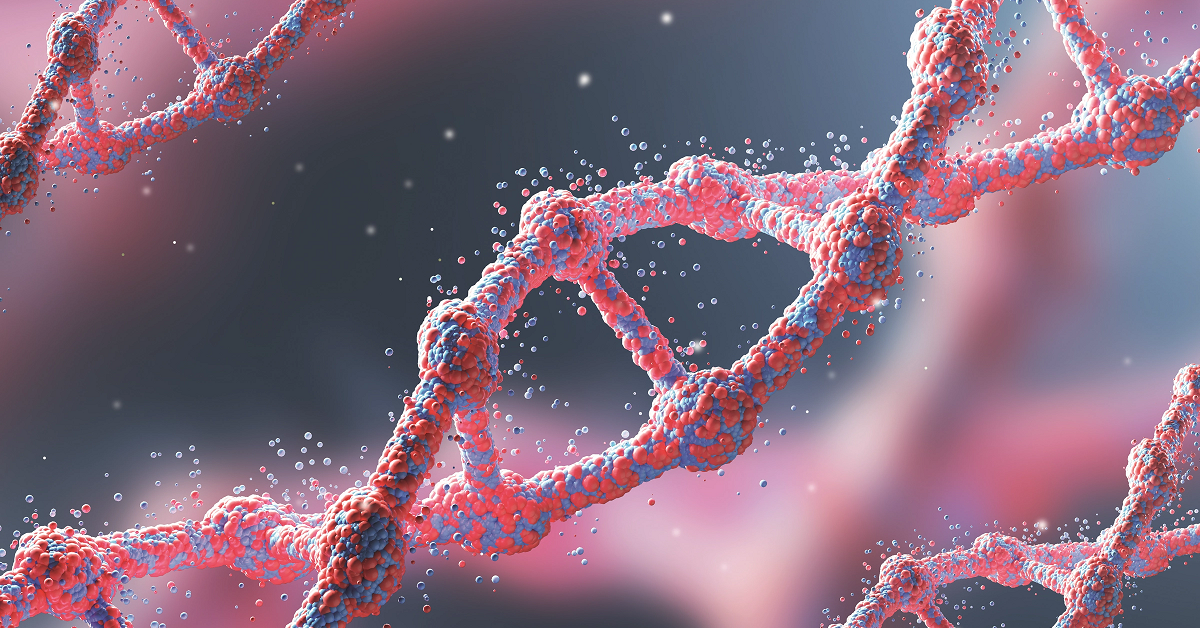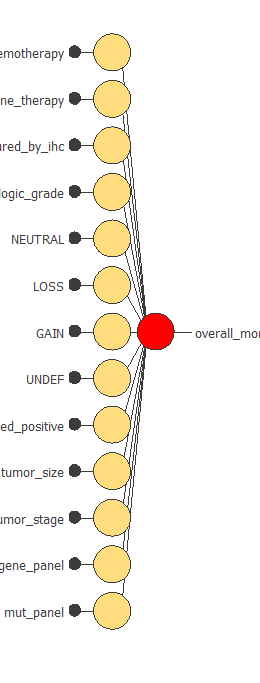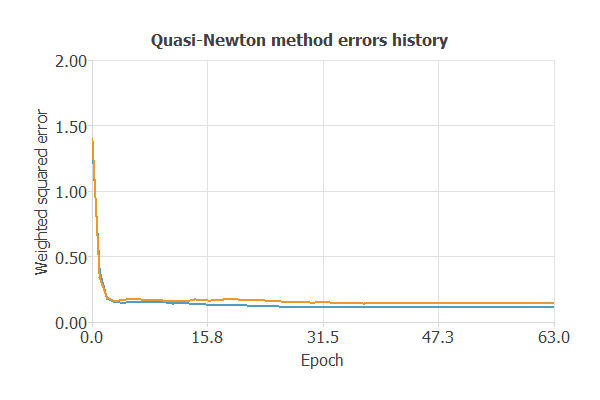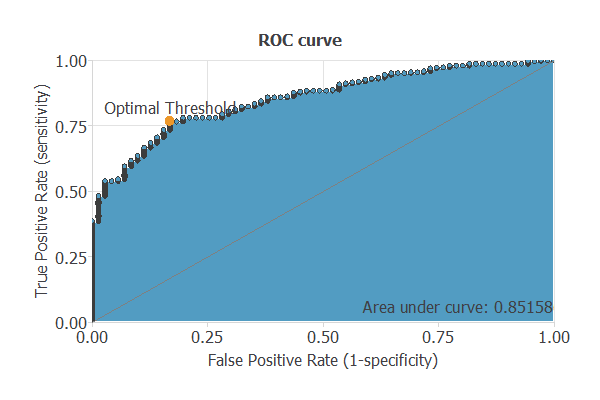# Breast cancer mortality prediction using machine learning

This example predicts the mortality of breast cancer patients over five years. We use different data types, including clinical and treatment variables, an expression, and a mutation panel. The genomic landscape of breast cancer is complex, and the heterogeneity in each case is a significant challenge in treating the disease.

The data are obtained from the Molecular Taxonomy of Breast Cancer International Consortium (METABRIC), a Canada-UK project containing targeted sequencing data from 1880 primary breast cancer patients. Clinical and genomic data were downloaded from cBioPortal.### Contents:

This example is solved with Neural Designer. You can follow it step by step using the free trial.

## 1. Application type

The predicted variable can have two values, "yes" if the patient has died and "no" otherwise. Therefore, this is a binary classification project.

This example aims to calculate a patient's risk of dying from breast cancer based on her clinical data, gene expression, and mutational profile using artificial intelligence and machine learning.

## 2. Data set

The 5_years_mortality.csv file contains the data for this example.

The number of rows in the data set is 1880. Each row corresponds to a different patient.

The number of columns is 688. Each column corresponds to 26 clinical variables where treatment is also included. In addition, an expression panel of 489 genes and a mutation panel of 173 genes.

The first 687 columns are the input variables.

After performing many simulations, the most optimal model obtained has the following variables:

• Chemotherapy: (1/0). Whether or not the patient had chemotherapy treatment.
• Hormone therapy:(1/0). Whether or not the patient had hormonal treatment.
• ER status measured by IHC: (1/0). Whether or not estrogen receptors are expressed in cancer cells by immunohistochemistry.
• Neoplasm histologic grade: (1, 2, 3). A description of a tumor is based on how abnormal the cancer cells and tissue look under a microscope and how quickly the cancer cells are likely to grow and spread. Low-grade cancer cells look more like normal cells and grow and spread more slowly than high-grade cancer cells.
• HER2 status measured by SNP6: (0, 1, -1). Whether or not the cancer is positive for HER2 using SNP microarrays.
• Lymph nodes examined positive: (0-45). Number of affected lymph nodes affected from original histopathology reports.
• PR status: (1/0). Whether or not the cancer cells are positive for progesterone receptors.
• Tumor size: (1-182). Tumor size measured by imaging techniques.
• Tumor stage: (0-4). The stage of cancer-based is on the involvement of surrounding structures, lymph nodes, and distant spread.
• Gene panel: 78 genes that contain m-RNA levels z-score.
• TP53 mutation: wether or not TP53 is mutated.

The last column is overall_mortality, the target variable. It has two values: 1 if the patient has died in the last five years or 0 otherwise.

The data set is divided into training, validation, and testing subsets. Neural Designer automatically assigns 60% of the instances for training, 20% for selection, and 20% for testing.

Once we have set the data, we can perform a few related analytics. We check the provided information and ensure that the data has good quality.

We can calculate the distributions for all variables. The following figure is a pie chart showing which patients had died in the data set.The image shows that patients who have died represent 18.5% of the samples, while 81.48% represent patients who live.

The inputs-targets correlations might indicate to us which factors most influence whether the mortality or the survival and therefore be more relevant to our analysis.The most correlated variables are Lymph nodes examined positive and Tumor stage. The inversely correlated variables are MAPT gene and ER status measured by IHC. It is known that the more affected the lymph nodes are, or the higher the level of staticity, the more likely it is that the disease will have a worse prognosis. On the other hand, ER-positive tumors are much more likely to respond to hormone therapy than ER-negative tumors. Therefore, the probability of death is lower.

## 3. Neural network

The next step is to set a neural network to represent the classification function. For this class of applications, the neural network is composed of:

The scaling layer contains the statistics on the inputs calculated from the data file and the method for scaling the input variables. Here the minimum-maximum method has been set. Nevertheless, the mean-standard deviation method would produce very similar results. The scaling layer has 90 inputs since 90 input variables are being used.

We won't use a perceptron layer to stabilize and simplify our model.

The probabilistic layer only contains the method for interpreting the outputs as probabilities. Moreover, as the output layer's activation function is the logistic, that output can already be interpreted as a probability of class membership. The probabilistic layer has one input. It has one output, representing the probability of a sample being a malignant tumor.

The following figure is a graphical representation of this neural network for breast cancer diagnosis.The yellow circles represent scaling neurons, and the red circles are probabilistic neurons. The number of inputs is 90, and the number of outputs is 1.

## 4. Training strategy

The fourth step is to set the training strategy, which is composed of two terms:

• A loss index.
• An optimization algorithm.

The loss index is the weighted squared error with L2 regularization. This is the default loss index for binary classification applications.

We can state the learning problem as finding a neural network that minimizes the loss index. That is, a neural network that fits the data set, error term, and does not oscillate, regularization term.

The optimization algorithm that we use is the quasi-Newton method. This is also the standard optimization algorithm for this type of problem.

The following chart shows how the error decreases with the iterations during the training process. The final training and selection errors are training error = 0.115 WSE and selection error = 0.143 WSE, respectively.## 5. Testing analysis

The objective of the testing analysis is to validate the performance of the generalization properties of the trained neural network. To validate a classification technique, we need to compare the values provided by this technique to the observed values. Therefore, we can use the ROC curve as it is the standard testing method for binary classification projects.A random classifier has an area under a curve of 0.5, while a perfect classifier has a value of 1. The closer the matter is to 1, the better the classifier. In this example, this parameter is AUC = 0.8516, which means a great performance.

The following table contains the elements of the confusion matrix. In addition, this matrix contains the true positives, false positives, false negatives, and true negatives for the variable diagnosis.

Predicted negative Predicted positive
Real positive 59 (16.8%) 12 (3.4%)
Real negative 66 (18.8%) 215 (61.1%)

The binary classification tests are parameters for measuring the performance of a classification problem with two classes:

• Classification accuracy (ratio of instances correctly classified): 77.8%
• Error rate (ratio of instances misclassified): 22.2%
• Sensitivity (ratio of real positive which are predicted positive): 83.09%
• Specificity (ratio of real negative which are predicted negative): 76.51%

## 6. Model deployment

Once we have tested the neural network's generalization performance, we can save it for future use with the model deployment function.

The mathematical expression represented by the neural network is written below.

```        scaled_chemotherapy = chemotherapy*(1+1)/(1-(0))-0*(1+1)/(1-0)-1;
scaled_hormone_therapy = hormone_therapy*(1+1)/(1-(0))-0*(1+1)/(1-0)-1;
scaled_er_status_measured_by_ihc = er_status_measured_by_ihc*(1+1)/(1-(0))-0*(1+1)/(1-0)-1;
scaled_NEUTRAL = NEUTRAL*(1+1)/(1-(0))-0*(1+1)/(1-0)-1;
scaled_LOSS = LOSS*(1+1)/(1-(0))-0*(1+1)/(1-0)-1;
scaled_GAIN = GAIN*(1+1)/(1-(0))-0*(1+1)/(1-0)-1;
scaled_UNDEF = UNDEF*(1+1)/(1-(0))-0*(1+1)/(1-0)-1;
scaled_lymph_nodes_examined_positive = (lymph_nodes_examined_positive-1.960680008)/3.98029995;
scaled_tumor_size = (tumor_size-26.05489922)/15.09500027;
scaled_tumor_stage = (tumor_stage-1.741140008)/0.5439749956;
scaled_bard1 = (bard1-0.229222998)/8.482979774;
scaled_mlh1 = (mlh1+0.5414069891)/8.592260361;
scaled_msh6 = (msh6-0.4801209867)/8.511969566;
scaled_ccne1 = (ccne1-1.780619979)/8.274580002;
scaled_cdk2 = (cdk2-0.3185670078)/8.434269905;
scaled_cdc25a = (cdc25a-1.122760057)/8.433389664;
scaled_ccnd1 = (ccnd1+0.1706919968)/8.495989799;
scaled_cdk6 = (cdk6-1.028929949)/8.539859772;
scaled_e2f4 = (e2f4-0.2499240041)/8.446029663;
scaled_e2f5 = (e2f5-0.5889610052)/8.618439674;
scaled_src = (src-0.4406799972)/8.487170219;
scaled_stat1 = (stat1-0.3746399879)/8.417449951;
scaled_stat5b = (stat5b+0.004886039998)/8.499250412;
scaled_cir1 = (cir1-0.1798630059)/8.481320381;
scaled_dll3 = (dll3-2.095230103)/8.162389755;
scaled_dtx3 = (dtx3-0.09693419933)/8.658659935;
scaled_kdm5a = (kdm5a-0.04170320183)/8.584600449;
scaled_notch1 = (notch1-0.4056940079)/8.542140007;
scaled_psen2 = (psen2-0.05174930021)/8.503979683;
scaled_hey2 = (hey2-1.119420052)/8.443579674;
scaled_aurka = (aurka-0.6015669703)/8.473730087;
scaled_bmpr1b = (bmpr1b-2.537029982)/7.147570133;
scaled_braf = (braf-0.7052929997)/8.568659782;
scaled_casp10 = (casp10+0.0159920007)/8.380310059;
scaled_casp3 = (casp3-0.2916800082)/8.576270103;
scaled_chek1 = (chek1-0.9011840224)/8.438759804;
scaled_dlec1 = (dlec1-1.021890044)/8.028869629;
scaled_eif4ebp1 = (eif4ebp1-1.375010014)/8.199110031;
scaled_erbb2 = (erbb2-1.260319948)/8.826080322;
scaled_erbb3 = (erbb3+0.6292200089)/8.349459648;
scaled_gsk3b = (gsk3b-0.4293929935)/8.670960426;
scaled_hif1a = (hif1a-0.2152210027)/8.391389847;
scaled_igf1r = (igf1r+0.3990350068)/8.577130318;
scaled_kras = (kras-0.4108310044)/8.67304039;
scaled_map2k4 = (map2k4-0.748036027)/8.610389709;
scaled_map3k1 = (map3k1-0.08064349741)/8.591899872;
scaled_map3k4 = (map3k4+0.2310950011)/8.441949844;
scaled_map3k5 = (map3k5-0.621776998)/8.469490051;
scaled_mmp12 = (mmp12-2.271709919)/7.930309772;
scaled_mmp7 = (mmp7-0.5178570151)/8.44064045;
scaled_mmp9 = (mmp9+0.183408007)/8.503649712;
scaled_nfkb1 = (nfkb1+0.06737440079)/8.420729637;
scaled_pdgfb = (pdgfb-0.8272690177)/8.535050392;
scaled_rheb = (rheb+0.1403409988)/8.75524044;
scaled_rps6kb2 = (rps6kb2-0.4955439866)/8.456430435;
scaled_slc19a1 = (slc19a1-0.5984209776)/8.261079788;
scaled_tgfb3 = (tgfb3+0.1925839931)/8.61067009;
scaled_gata3 = (gata3+1.651250005)/8.173270226;
scaled_runx1 = (runx1-0.2606729865)/8.459420204;
scaled_tbx3 = (tbx3-0.1103060022)/8.374890327;
scaled_abcc10 = (abcc10-0.6760720015)/8.525839806;
scaled_map2 = (map2-1.531759977)/8.391799927;
scaled_mapt = (mapt+0.900497973)/8.5144701;
scaled_tubb4b = (tubb4b-0.06924349815)/8.584989548;
scaled_ahnak = (ahnak+0.5138469934)/8.548529625;
scaled_arid2 = (arid2-0.004590429831)/8.589099884;
scaled_chd1 = (chd1-0.2260349989)/8.42580986;
scaled_fancd2 = (fancd2-0.4411639869)/8.524129868;
scaled_flt3 = (flt3-1.482869983)/8.095809937;
scaled_lama2 = (lama2-0.4525449872)/8.49695015;
scaled_ncoa3 = (ncoa3-0.4167970121)/8.499380112;
scaled_nek1 = (nek1-0.1626999974)/8.533590317;
scaled_nr3c1 = (nr3c1+0.1318700016)/8.444359779;
scaled_nras = (nras-0.4719530046)/8.548589706;
scaled_prkcq = (prkcq-0.07657650113)/8.548060417;
scaled_rpgr = (rpgr+0.1501130015)/8.611829758;
scaled_siah1 = (siah1-0.03885160014)/8.571940422;
scaled_ar = (ar+0.8043000102)/8.666520119;
scaled_cdk8 = (cdk8-0.3470639884)/8.561869621;
scaled_cyp21a2 = (cyp21a2-0.7355030179)/8.248609543;
scaled_hes6 = (hes6-0.6975460052)/8.417490005;
scaled_hsd17b2 = (hsd17b2-2.470020056)/7.934319973;
scaled_hsd17b8 = (hsd17b8+0.3868130147)/8.583809853;
scaled_nrip1 = (nrip1-0.1014230028)/8.576760292;
scaled_serpini1 = (serpini1-1.421460032)/8.006059647;
scaled_srd5a3 = (srd5a3-0.4584310055)/8.268830299;
scaled_tp53_mut = tp53_mut*(1+1)/(1-(0))-0*(1+1)/(1-0)-1;

probabilistic_layer_combinations_0 = -0.994578 +0.169719*scaled_chemotherapy -0.102114*scaled_hormone_therapy -0.027081*scaled_er_status_measured_by_ihc +0.0706069*scaled_neoplasm_histologic_grade +0.220035*scaled_NEUTRAL +0.528728*scaled_LOSS +0.214109*scaled_GAIN +1.0203*scaled_UNDEF +0.306879*scaled_lymph_nodes_examined_positive +0.233669*scaled_tumor_size +0.278039*scaled_tumor_stage +0.125232*scaled_bard1 -0.0215592*scaled_mlh1 +0.193355*scaled_msh6 +0.146971*scaled_ccne1 -0.142463*scaled_cdk2 -0.0349746*scaled_cdc25a +0.0890444*scaled_ccnd1 -0.00491231*scaled_cdk6 +0.179044*scaled_e2f4 +0.0535495*scaled_e2f5 -0.300746*scaled_src -0.0390889*scaled_stat1 -0.127806*scaled_stat5b +0.108092*scaled_adam17 -0.160666*scaled_cir1 +0.0437408*scaled_dll3 +0.103283*scaled_dtx3 -0.119082*scaled_kdm5a -0.199069*scaled_notch1 +0.0230085*scaled_psen2 +0.0613692*scaled_hey2 +0.0811372*scaled_aurka +0.00713841*scaled_bmpr1b -0.114506*scaled_braf +0.206722*scaled_casp10 +0.0947801*scaled_casp3 +0.187272*scaled_chek1 -0.0852123*scaled_dlec1 +0.0666705*scaled_eif4ebp1 +0.107631*scaled_erbb2 +0.0125677*scaled_erbb3 +0.092772*scaled_gsk3b +0.112979*scaled_hif1a +0.0651637*scaled_igf1r -0.00769582*scaled_kras +0.0396564*scaled_map2k4 +0.0216436*scaled_map3k1 +0.407818*scaled_map3k4 -0.0899417*scaled_map3k5 -0.13832*scaled_mmp12 -0.12894*scaled_mmp7 +0.0104902*scaled_mmp9 -0.286661*scaled_nfkb1 +0.187826*scaled_pdgfb -0.0735231*scaled_rheb +0.104951*scaled_rps6kb2 +0.102162*scaled_slc19a1 +0.27306*scaled_smad6 -0.056902*scaled_tgfb3 -0.177689*scaled_gata3 -0.00270286*scaled_runx1 +0.023676*scaled_tbx3 -0.0765527*scaled_abcc10 -0.194937*scaled_map2 -0.0765514*scaled_mapt -0.0719315*scaled_tubb4b +0.258059*scaled_ahnak -0.133846*scaled_arid2 +0.00883793*scaled_chd1 +0.0768032*scaled_fancd2 -0.11919*scaled_flt3 +0.0778854*scaled_lama2 +0.056394*scaled_ncoa3 +0.00449616*scaled_nek1 -0.0817723*scaled_nr3c1 -0.00223693*scaled_nras -0.0964826*scaled_prkcq +0.0286699*scaled_rpgr +0.0676205*scaled_siah1 -0.0976069*scaled_ar +0.100766*scaled_cdk8 -0.0404574*scaled_cyp21a2 -0.158643*scaled_hes6 +0.0346541*scaled_hsd17b2 -0.162662*scaled_hsd17b8 +0.0676852*scaled_nrip1 -0.0558861*scaled_serpini1 +0.0512602*scaled_srd5a3 +0.2682*scaled_tp53_mut

overall_mortality = 1.0/(1.0 + exp(-probabilistic_layer_combinations_0);
```

The above expression can be exported anywhere, for instance, to a dedicated diagnosis software used by doctors. It can even be integrated into a website:

Please note that it is impossible to predict the future with certainty, and a physician must always interpret these predictions to make a diagnosis.

## Conclusions

Combining clinical variables, gene expression, and mutation profiles provide a richer understanding of breast cancer's genomic landscape and offer new insights into inter and intra-tumor heterogeneity that should inform the future development of clinical management of patients.

## Related posts:The development of this application has been funded by the NEMHESYS - NGS Establishment in Multidisciplinary Healthcare Education System project.